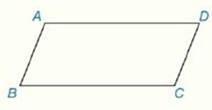Chapter 4.CR, Problem 14CRElementary Geometry For College St...

7th Edition
Alexander + 2 others
ISBN: 9781337614085

Solutions

Chapter
SectionElementary Geometry For College St...

7th Edition
Alexander + 2 others
ISBN: 9781337614085
Textbook Problem

Review Exercises Given: ▱ A B C D m ∠ A = 2 x + 6 m ∠ B = x + 24 Find:   m ∠ CTo determine

To Find:

The measure of angle C of the parallelogram ABCD with the help of provided information.

Explanation

Properties Used:

1. Two consecutive angles of a parallelogram are supplementary.

2. The opposite sides of a parallelogram are congruent.

Calculation:

It is given that ABCD is a parallelogram with mA=2x+6 and mB=x+24.

In the parallelogram ABCD, mA and mB are supplementary.

That is, mA+mB=180°.

Applying the values in the above supplementary formula,

2x+6+x+24=180°

3x+30=180°

3<

Still sussing out bartleby?

Check out a sample textbook solution.

See a sample solution

The Solution to Your Study Problems

Bartleby provides explanations to thousands of textbook problems written by our experts, many with advanced degrees!

Get Started

Fill in each blank: 6gal3qt=pat

Elementary Technical Mathematics

What are the two requirements for a random sample?

Statistics for The Behavioral Sciences (MindTap Course List)

Find f. f(x) = 4 + 6x + 24x2, f(0) = 3, f(1) = 10

Single Variable Calculus: Early Transcendentals, Volume I

True or False: n=1(1)nn+14 is a convergent series.

Study Guide for Stewart's Single Variable Calculus: Early Transcendentals, 8th